I find histograms to be fascinating—both in the insights that they can bring us and in the various complexities we run into when creating them in Tableau. If you’re a regular reader of our blog or you happened to catch Kevin and I at Tableau Conference 2022, you may remember that I’ve addressed this topic in the past. In our TC22 presentation and in How to Build Lovely Histograms in Tableau, I provided some of my favorite tips for building nice histograms in Tableau. But that blog glossed over something that probably deserves a bit more attention—the bin size. Instead of letting Tableau choose a bin size for me, I created a parameter so that users can choose their own bin size. I like this technique as it allows the user to explore the data by experimenting with different bin sizes. But there are some instances where you’d be better off setting the bin size yourself or using something more dynamic and not letting the users touch it. For instance, perhaps your users are new to data visualization and have no idea what bin size to choose? Or what if you apply filters to your chart, which causes those bin sizes to be nonsensical? Or what if your data changes significantly over time, making the bin sizes inapplicable?

Let’s look at an example. Below I’ve created a histogram showing the number of rows binned by sales. I’m using a parameter value of 400 for the bin size.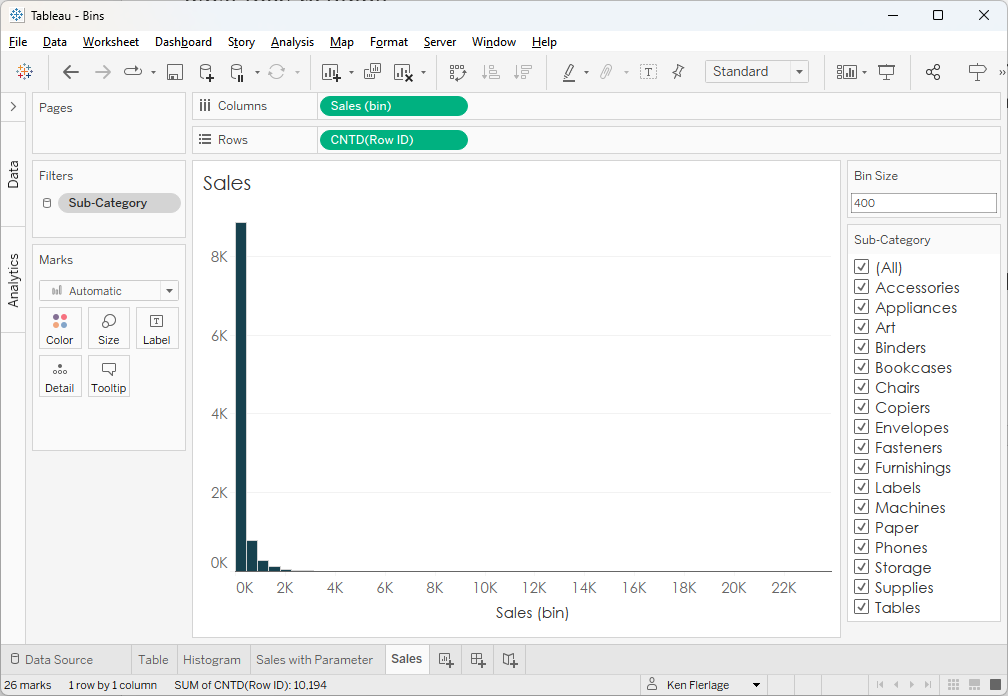When we’re looking at all sub-categories, this bin size might make sense, but what if we filter down to a specific sub-category? For example, let’s look at Envelopes.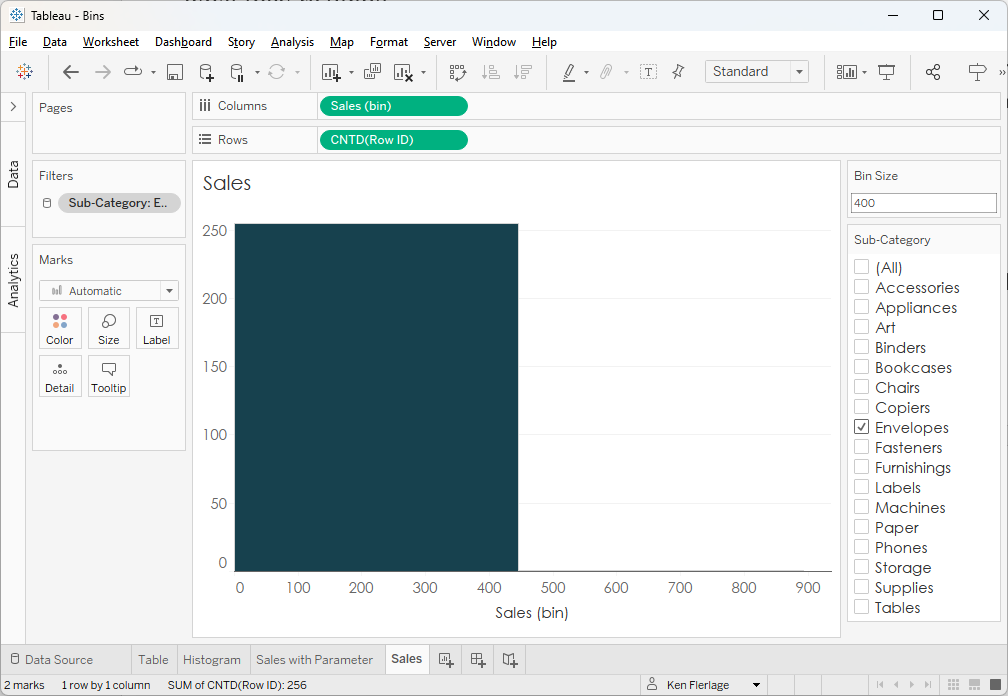Envelopes are comparatively cheap, so it’s not surprising that sales of envelopes almost always fall within that first bin of 0-400. In this case, it might be better to choose a bin size that is more applicable to the data in our view. With the bin size parameter, our users can change it manually, but if they are filtering a lot, the constant editing of the bin size could be a burden—especially if our users aren’t quite sure what they’re doing.

So, in these situations, it might be better for us to create a dynamic bin size that automatically adjusts based on the data in our view. This is the exact problem I recently addressed on a post on the Community Forums. And, today, I’ll share a technique for auto-adjusting dynamic bins in Tableau.

Calculating the Bin Size

OK, so let’s give this a try and see what we can do. We could, of course, just let Tableau choose the bin size for us. So, let’s create bins on Sales…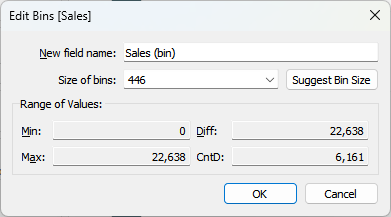Tableau automatically chooses a bin size of 446 for us. Can you see the problem already? It’s creating this value by looking at the entire data set and then it’s setting that as a static bin size. But tomorrow your data might completely change, making this value no longer applicable. Or, as we showed earlier, your users might filter the data, limiting the usefulness of this static value. So, how can we can choose the value Tableau suggests but also make it dynamic?

Well, if we knew the math that Tableau uses to calculate the bin size, then we might be able to calculate it dynamically, right? The good news is that the math Tableau uses is known. Jeffrey Shaffer’s blog, Bin There, Done That: A Discussion of Bins in Tableau gives us the exact math:

Bin Size = (Max ValueMin Value)/Number of Bins

Number of Bins = 3 + log2(n) * log(n)

…where n is the unique number of values in that measure.

Let’s do this in small chunks of calculated fields. We’ll start by counting n—the number of unique values in our measure.

1. Distinct Values

// Number of unique values of the measure.

COUNTD([Sales])

Now, since we have the value of n, we can calculate the number of bins.

2. Number of Bins

// Formula to get number of bins is 3 + log2(n) * log(n)

// log2(n) = log(n)/log(2).

3 + LOG([1. Distinct Values])/LOG(2) * LOG([1. Distinct Values])

Next, we can find the min and max values of the measure, then find the difference.

3. Min Value

// Minimum value of profit.

MIN([Sales])

4. Max Value

// Maximum value of profit.

MAX([Sales])

5. Difference

// Difference between min and max.

[4. Max Value]-[3. Min Value]

Then we can apply the Bin Size Calculation

6. Bin Size

// Bin size = (Max Value - Min Value)/Number of Bins

// Use LOD to make sure this value works across all levels of detail.

{FIXED : [5. Difference]/[2. Number of Bins]}

Now let’s look at the value of each of these variables.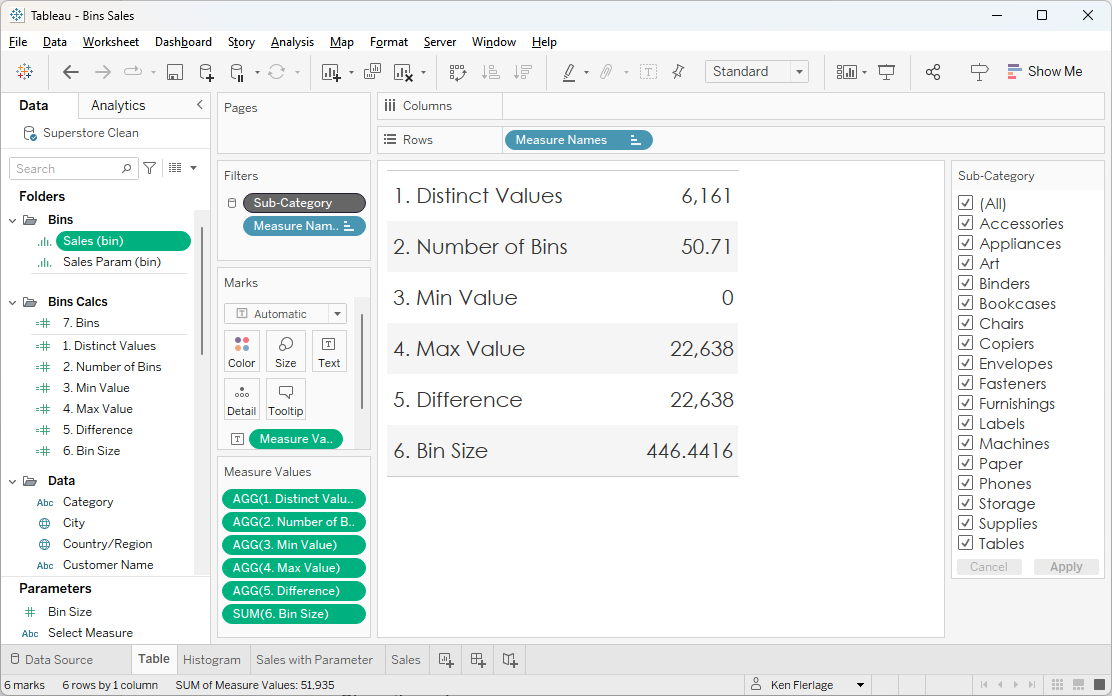As we can see here, our bin size is approximately 446. That should look familiar as it matches the bin size that Tableau chose for us automatically. That’s great news as it validates our calculations! However, unlike the number Tableau chose for us, this value is now dynamic and will adjust to the data in our view. So, if we filter to Envelopes, we’ll get a different bin size.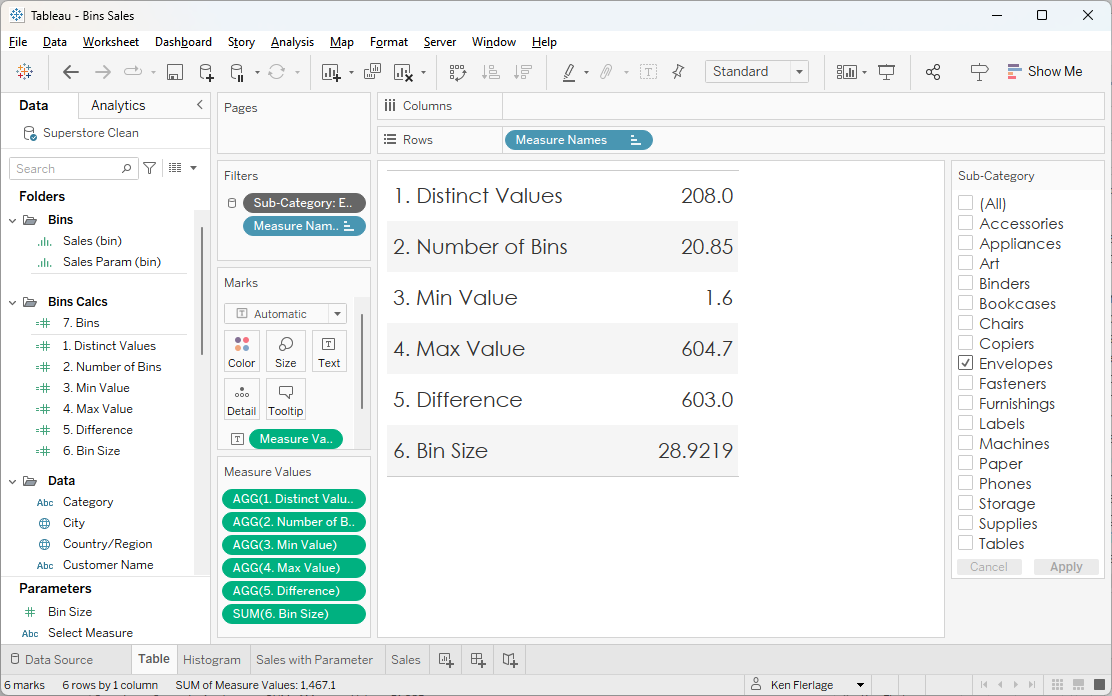Note: Since the Bin Size calculated field uses an LOD, the filter on Sub-Category has been changed to a context filter so that it computes before the LOD in the Order of Operations.

As we can see, we now get a much smaller bin size of approximately 29.

Using it with Our Bins

Now that we have the bin size, we need to use it in our bins. But we immediately run into a problem. Built-in Tableau bins only give you two options—a static value or a parameter. Unfortunately, neither of those options will allow us to use a calculated field. We could tinker with dynamic parameters, but those only update when the workbook is opened, so I guess we’re just stuck—but hey, it was a good effort, right? Of course not!! As detailed on my other histograms post, we can leverage the “Bring Your Own Bins” technique developed by Joe Mako, which I first learned about from Jonathan Drummey’s blog. This technique entails the use of a relatively simple calculated field instead of built-in bins. The calc looks like this:

INT([Value]/[Bin Size])*[Bin Size]-IIF([Value]<0,[Bin Size],0)

Where Value is our measure and Bin Size, of course, is our bin size. Using Sales as our measure, we now have this:

7. Bins

INT([Sales]/[6. Bin Size])* [6. Bin Size]-IIF([Sales]<0, [6. Bin Size],0)

Now we can use this instead of our built-in bins field:

Note: Be sure to apply some of the techniques addressed in my other blog, including dropping Bin Size on the size card and changing the mark type to bar.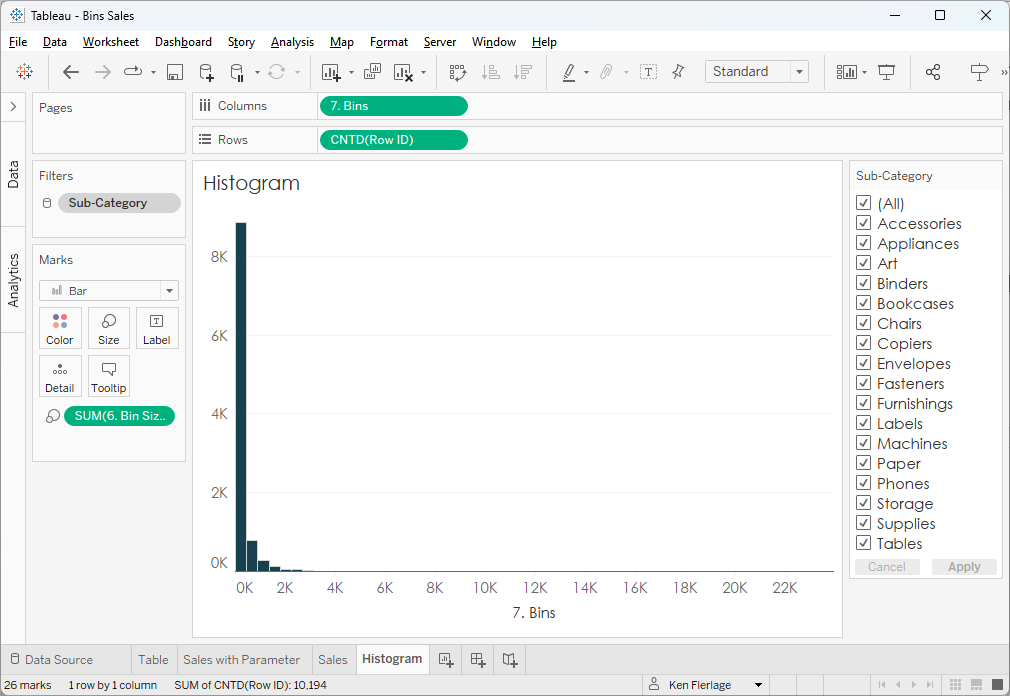Now we have something very similar to what we created at the very beginning. However, as data changes or we change filters, the bin size will automatically adjust. Let’s select Envelopes to see what happens.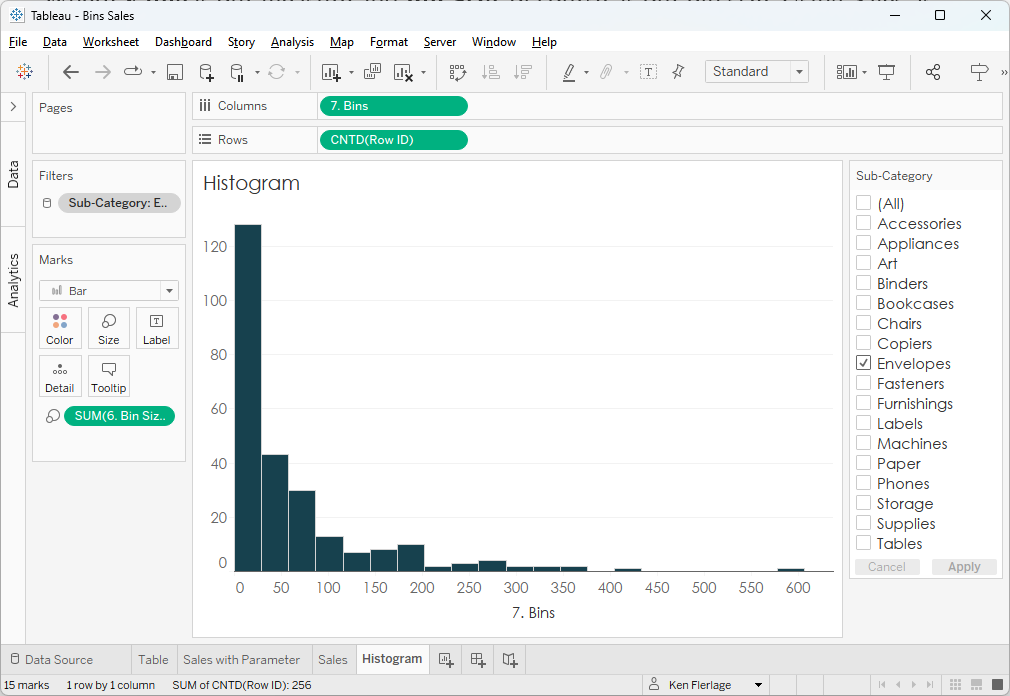Our bin size has now adjusted to 29, giving us a histogram that makes much more sense when only viewing Envelopes than the one with a bin size of 446.

Wrap-Up

I personally had a lot of fun working through this problem. While this blog was definitely quite wonky, I think that there are many scenarios where this technique makes a lot more sense than choosing the initial recommended bin size or using a parameter. It may not be the best solution in all cases, but I’m hopeful that it will come in handy in a variety of situations.

Thanks for reading. If you have any questions or thoughts, please feel free to leave them in the comments section below.

Ken Flerlage, June 26, 2023